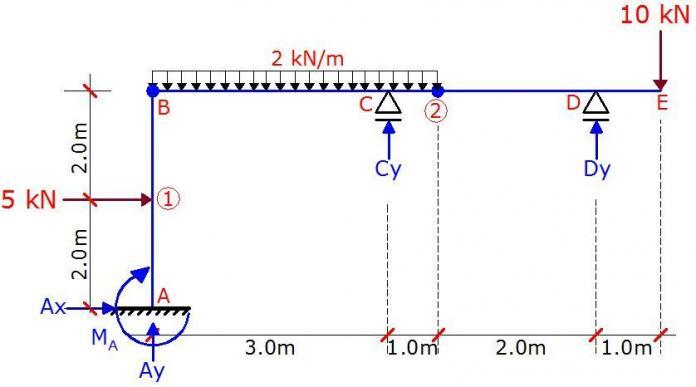# Analysis of Statically Determinate Compound Frames (Part 3): A solved ExampleQuestion
A frame is loaded as shown above. There are internal hinges at nodes B and 2. Plot the internal stresses diagram due to the externally applied load.

Solution
Check for static determinacy.
A quick but non-general way of verifying degree of static indeterminacy of simple frames is;

D = R – e – S

where;
D = Degree of static indeterminacy
R = Number of support reactions = 5
e = Number of static equilibrium equations = 3
S = Number of special condition = 2 ( internal hinges)

D = 5 – 3 – 2 = 0
Therefore, the structure is statically determinate and stable.

Static Determinacy of Rigid Frames
Understanding Sign Conventions in Structural Analysis

Support Reactions
Let ∑FX = 0
Ax + 5 = 0
Ax = -5 KN ←

Let ∑M2R = 0
2Dy – (10 × 3) = 0
Dy = 15 KN

Let ∑MBR = 0
6Dy + 3Cy – (10 × 7) – ((2 × 42)/2) = 0
6(15) + 3Cy – 70 – 16 = 0
Cy = -1.333KN↓

Let ∑MBB = 0
Note that the due to the direction of the horizontal reaction A, it will exert a positive moment about column AB. Therefore;
4Ax – (5 × 2) – MA = 0
4(5) – 10 = MA
MA = 10 KN.m

Let ∑M2L = 0
4Ay + 4Ax + Cy – MA – ((2 × 42)/2) – (5 × 2) = 0
4Ay + 4(5) – 1.333 – 10 – 16 – 10 = 0
Ay = 4.333 KN

Equilibrium check;
∑FY ↓ = (2 × 8) + 10 = 18KN
∑FY ↑ = 15 – 1.333 + 4.333 = 18KN OKFig 1: Support Reactions Due to Externally Applied Load

Analysis of Internal forces

Section A – 1 (0 ≤ y ≤ 2.0m)
(i) Bending Moment
My = 5y – 10 ———— (1)
At y = 0;
MA = -10 kNm
At y = 2.0m;
M1B = 5(2) – 10 = 0

(ii) Shear Force
The section is subjected to constant shear force;
Qx = ∂My/∂y = 5 KN

(iii) Axial Force
Ny + 4.333 = 0
NA-1 = -4.333 KN (compression)

Section 1 – B (2.0m ≤ y ≤ 4.0m)
(i) Bending Moment
My = 5y – 5(y – 2) – 10 ———— (2)
At y = 2m;
M1UP = 5(2) – 10 = 0
At y = 4.0m;
MBB = 5(4) – 5(2) – 10 = 0
This shows that there is no bending moment at this section.

(ii) Shear Force
Differentiating equation (1) above yields zero. Therefore, there is shear force at the section also.

(iii) Axial Force
Ny + 4.333 = 0
N1-B = -4.333 KN (compression)

Section B – C (0 ≤  x ≤ 3.0m)
(i) Bending Moment
Mx = 4.333xx2 ———— (3)
At x = 0;
MBR = 0
At x = 3.0m;
MCL = 4.333(3) – (3)2 = 4 KNm

Maximum moment occurs at the point of zero shear. Therefore;
Qx = ∂Mx/∂x = 4.333 – 2x = 0
On solving;
x = 4.333/2 = 2.1665m
Mmax = 4.333(2.1665) – (2.1665)2 = 4.693 KNm

(ii) Shear Force
The section is subjected to constant shear force;
Qx = ∂Mx/∂x = 4.333 – 2x

At x = 0;
QBR = 4.333 KN
At x = 3.0m;
MCL = 4.333 – 2(3) = -1.667 KN

(iii) Axial Force
Nx = 0
No axial force

Section C – 2 (3.0m ≤ x ≤ 4.0m)
(i) Bending Moment
Mx = 4.333x – 1.333(x – 3) – x2 ———— (4)
At x = 3m;
MCR = 4.333(3) – 32 = 4 KNm
At x = 4.0m;
M2 = 4.333(4) – 1.333(1) – (4)2 = 0

(ii) Shear force
Expanding equation (4) above;
Mx = –x2 + 3x + 4

Qx = ∂Mx/∂x = -2x + 3

At x = 3m
QCR = -2(3) + 3 = -3 KN
At x = 4m
Q2L = -2(4) + 3 = -5 KN

(iii) Axial Force
No axial force

Section D – E (0 ≤  x  ≤ 1.0)
(i) Bending Moment
Mx = -10x ————— (5)

At x = 0;
ME = 0
At x = 1.0m;
MCL = -10(1) = -10 KNm

(ii) Shear Force
Qx = ∂Mx/∂x = -10 KN

But since we are coming from the right to the left, downward force is positive.
Therefore the section subjected to a positive constant shear force of 10 KN

(iii) Axial force
There is no axial force at the section

Internal Stresses Diagram
We can now plot the internal stresses diagram from the values obtained above.Fig 2: Final Bending Moment DiagramFig 3: Final Shear Force DiagramFig 4: Final Axial Force Diagram

Thank you for visiting Structville today.
1.Celestine Zimbi